Скачать презентацию Higher Derivatives Concavity 2 nd Derivative Test Lesson

7e71d473e62d8e00a0646d5d42599b20.ppt

• Количество слайдов: 13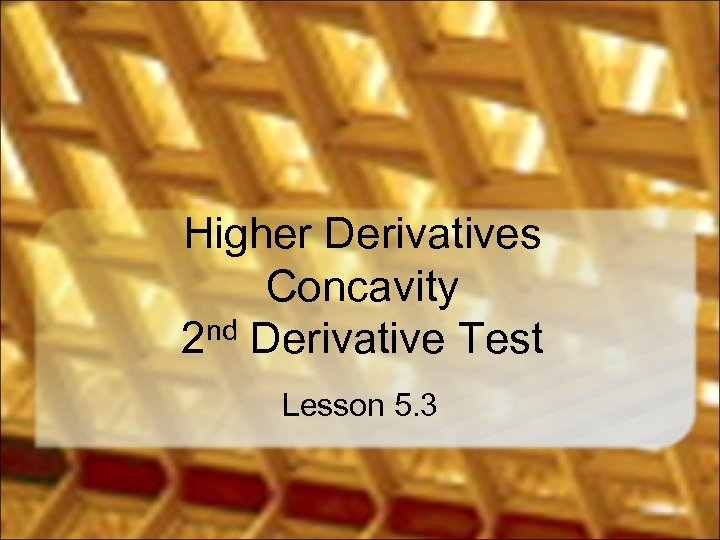Higher Derivatives Concavity 2 nd Derivative Test Lesson 5. 3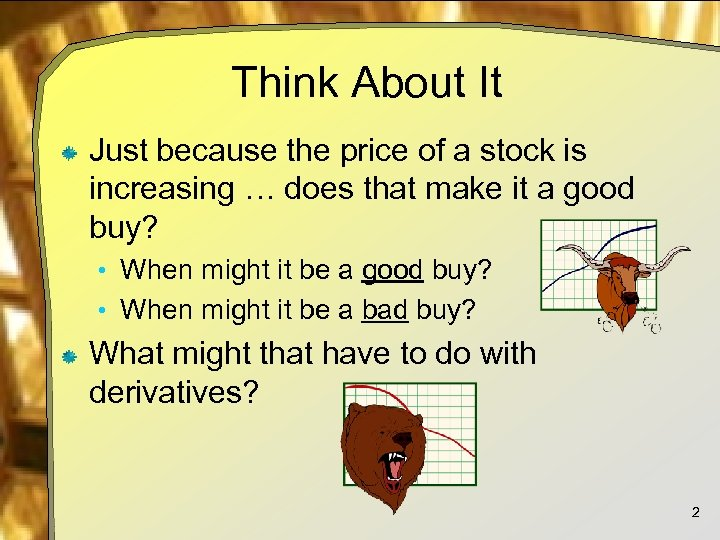Think About It Just because the price of a stock is increasing … does that make it a good buy? • When might it be a bad buy? What might that have to do with derivatives? 2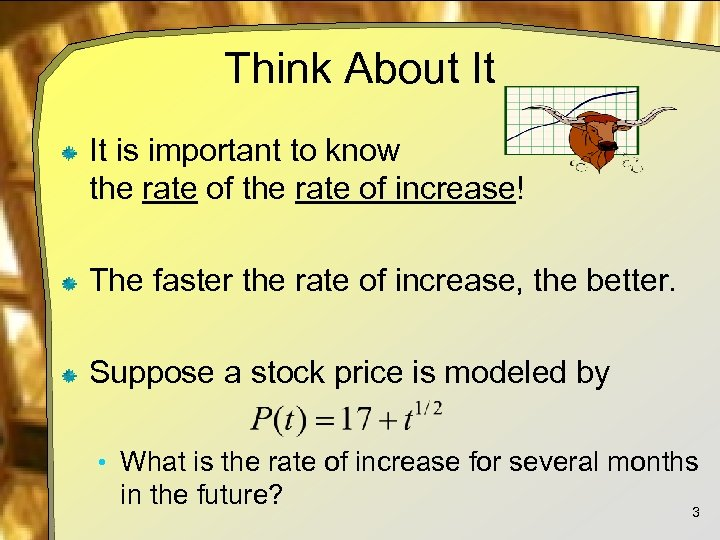Think About It It is important to know the rate of increase! The faster the rate of increase, the better. Suppose a stock price is modeled by • What is the rate of increase for several months in the future? 3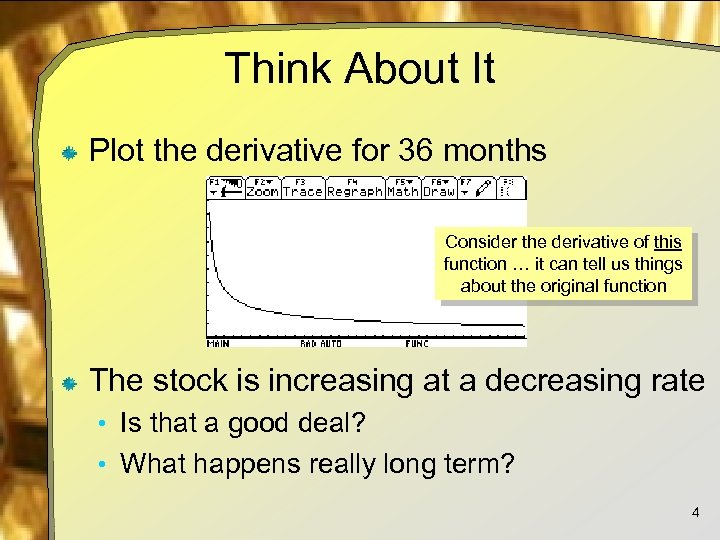Think About It Plot the derivative for 36 months Consider the derivative of this function … it can tell us things about the original function The stock is increasing at a decreasing rate • Is that a good deal? • What happens really long term? 4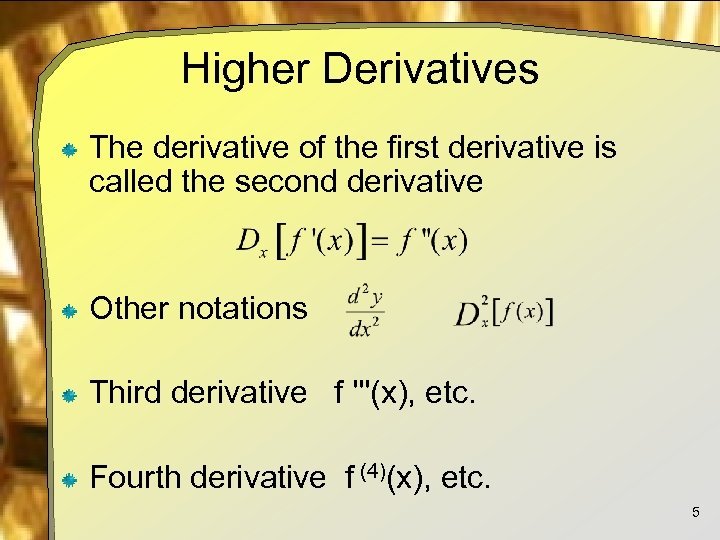Higher Derivatives The derivative of the first derivative is called the second derivative Other notations Third derivative f '''(x), etc. Fourth derivative f (4)(x), etc. 5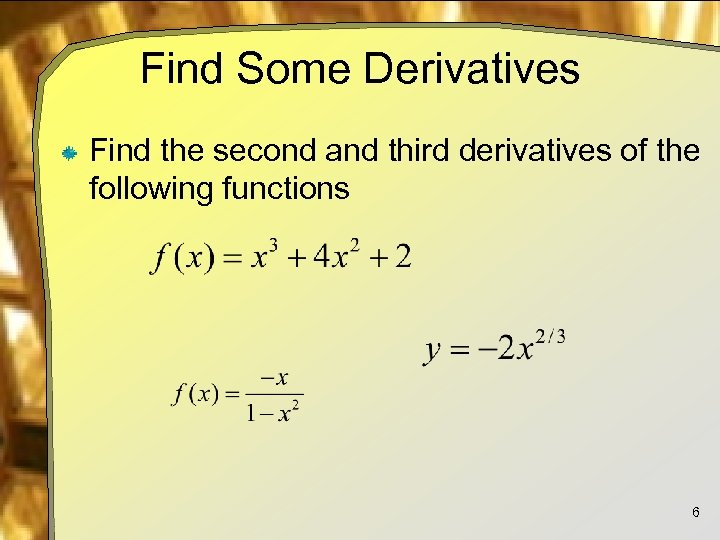Find Some Derivatives Find the second and third derivatives of the following functions 6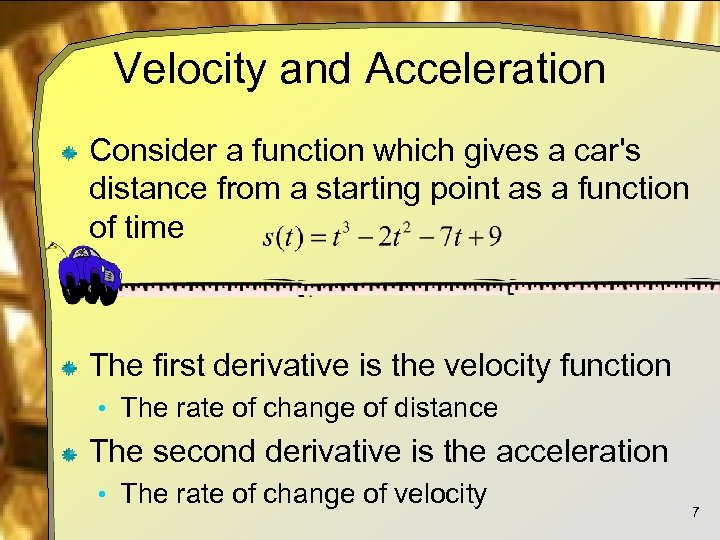Velocity and Acceleration Consider a function which gives a car's distance from a starting point as a function of time The first derivative is the velocity function • The rate of change of distance The second derivative is the acceleration • The rate of change of velocity 7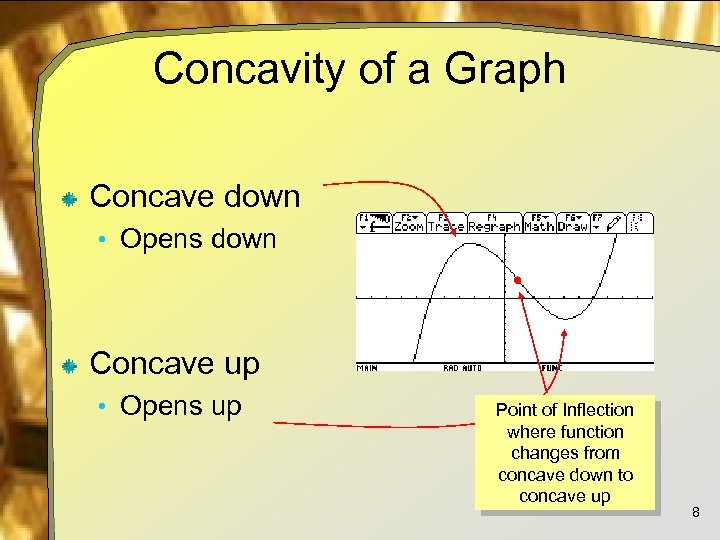Concavity of a Graph Concave down • Opens down Concave up • Opens up Point of Inflection where function changes from concave down to concave up 8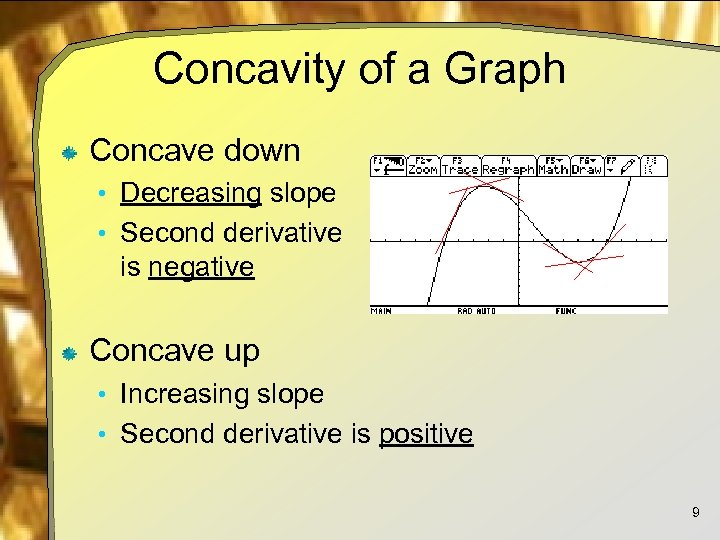Concavity of a Graph Concave down • Decreasing slope • Second derivative is negative Concave up • Increasing slope • Second derivative is positive 9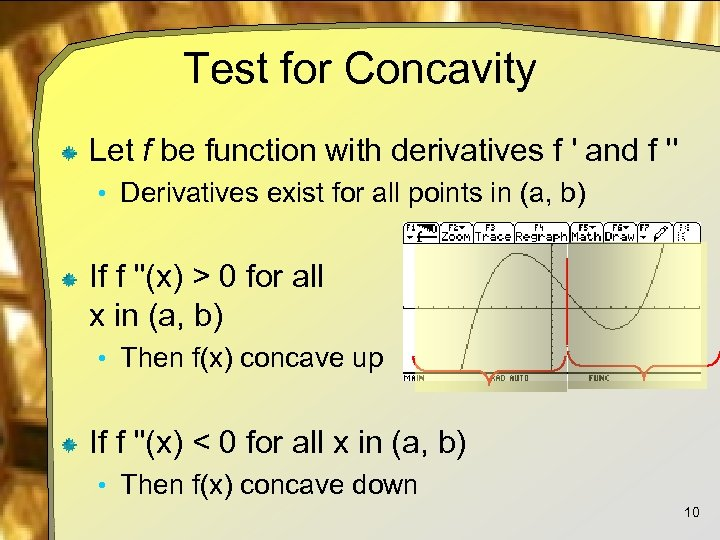Test for Concavity Let f be function with derivatives f ' and f '' • Derivatives exist for all points in (a, b) If f ''(x) > 0 for all x in (a, b) • Then f(x) concave up If f ''(x) < 0 for all x in (a, b) • Then f(x) concave down 10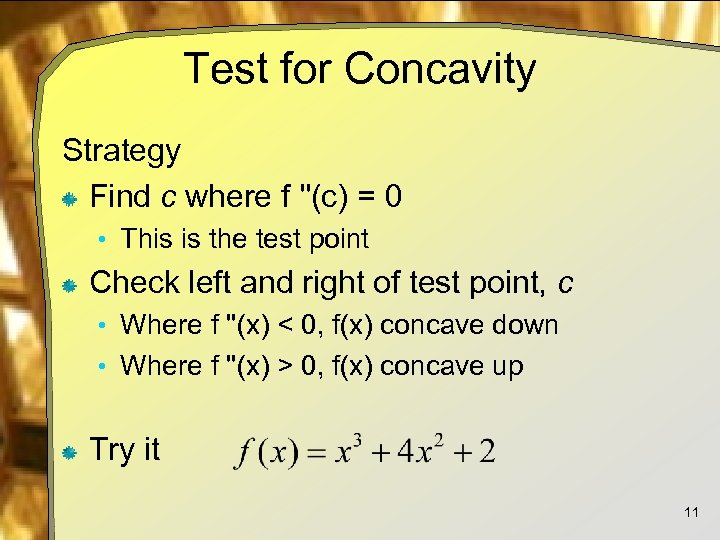Test for Concavity Strategy Find c where f ''(c) = 0 • This is the test point Check left and right of test point, c • Where f ''(x) < 0, f(x) concave down • Where f ''(x) > 0, f(x) concave up Try it 11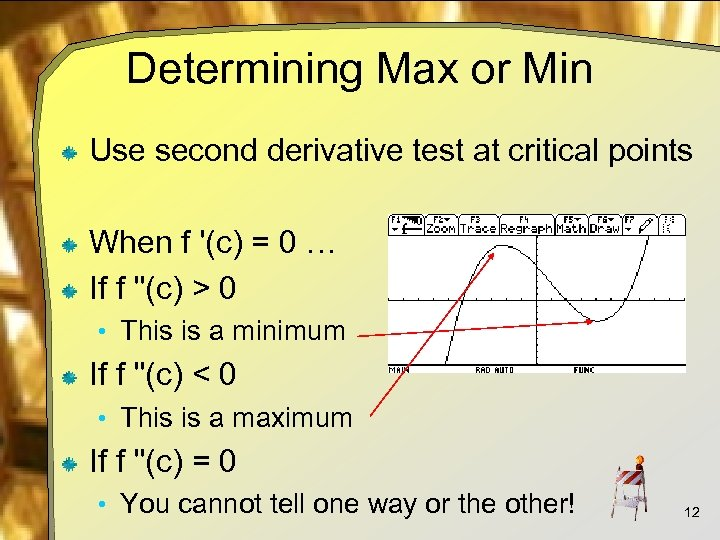Determining Max or Min Use second derivative test at critical points When f '(c) = 0 … If f ''(c) > 0 • This is a minimum If f ''(c) < 0 • This is a maximum If f ''(c) = 0 • You cannot tell one way or the other! 12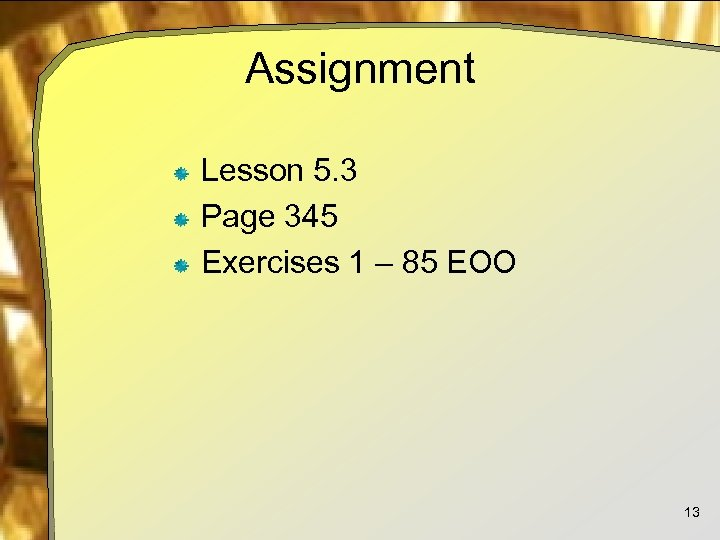Assignment Lesson 5. 3 Page 345 Exercises 1 – 85 EOO 13